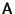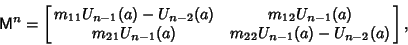## Unimodular Matrix

A Matrixwith Integer elements and Determinant det± 1, also called a Unit Matrix.

The inverse of a unimodular matrix is another unimodular matrix. A Positive unimodular matrix has det. Theth Power of a Positive Unimodular Matrix(1)

is(2)

where(3)

and theare Chebyshev Polynomials of the Second Kind,(4)